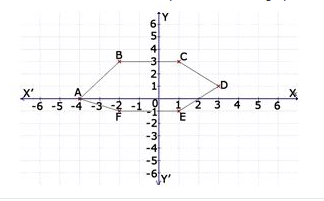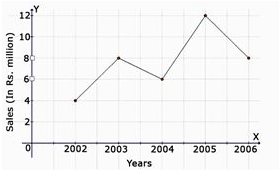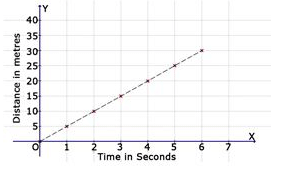Test: Introduction To Graphs- 2

# Test: Introduction To Graphs- 2

Test Description

## 20 Questions MCQ Test Mathematics (Maths) Class 8 | Test: Introduction To Graphs- 2

Test: Introduction To Graphs- 2 for Class 8 2023 is part of Mathematics (Maths) Class 8 preparation. The Test: Introduction To Graphs- 2 questions and answers have been prepared according to the Class 8 exam syllabus.The Test: Introduction To Graphs- 2 MCQs are made for Class 8 2023 Exam. Find important definitions, questions, notes, meanings, examples, exercises, MCQs and online tests for Test: Introduction To Graphs- 2 below.
Solutions of Test: Introduction To Graphs- 2 questions in English are available as part of our Mathematics (Maths) Class 8 for Class 8 & Test: Introduction To Graphs- 2 solutions in Hindi for Mathematics (Maths) Class 8 course. Download more important topics, notes, lectures and mock test series for Class 8 Exam by signing up for free. Attempt Test: Introduction To Graphs- 2 | 20 questions in 20 minutes | Mock test for Class 8 preparation | Free important questions MCQ to study Mathematics (Maths) Class 8 for Class 8 Exam | Download free PDF with solutions
 1 Crore+ students have signed up on EduRev. Have you?
Test: Introduction To Graphs- 2 - Question 1

### Find the coordinates of the point C from the graph.Detailed Solution for Test: Introduction To Graphs- 2 - Question 1

As x-coordinate is 1 unit and y-coordinate is 3 units.

Test: Introduction To Graphs- 2 - Question 2

### Find the coordinates of the point D from the graph.Test: Introduction To Graphs- 2 - Question 3

### Find the coordinates of the point E from the graph.Test: Introduction To Graphs- 2 - Question 4

Find the coordinates of the point F from the graph.Test: Introduction To Graphs- 2 - Question 5

In which quadrant does the point Q (-2, -6) lie?

Detailed Solution for Test: Introduction To Graphs- 2 - Question 5

The third quadrant is the quadrant where x and y are negative. Hence (-2,-6) lie in 3rd quadrant

Test: Introduction To Graphs- 2 - Question 6

(o, y) are the co-ordinates of a point lying on which of the following?

Detailed Solution for Test: Introduction To Graphs- 2 - Question 6

As we don’t have any units for x-coordinate the ,so the point lies on the y-axis.

Test: Introduction To Graphs- 2 - Question 7

The line graph shows the yearly sales figure for a manufacturing company. From the graph, what were the sales in 2004?Test: Introduction To Graphs- 2 - Question 8

The line graph shows the yearly sales figure for a manufacturing company. From the graph, what were the sales in 2002?Test: Introduction To Graphs- 2 - Question 9

The line graph shows the yearly sales figure for a manufacturing company. From the graph, what were the sales in 2003?Test: Introduction To Graphs- 2 - Question 10

The line graph shows the yearly sales figure for a manufacturing company. From the graph, what were the sales in 2005?Test: Introduction To Graphs- 2 - Question 11

The line graph shows the yearly sales figure for a manufacturing company. From the graph, what were the sales in 2006?Test: Introduction To Graphs- 2 - Question 12

On which axis does the point (0, 5) lie?

Detailed Solution for Test: Introduction To Graphs- 2 - Question 12

Solution :- The point (0,5) lies on Y- axis.

Hence, x=0, y=5

Test: Introduction To Graphs- 2 - Question 13

In which quadrant does the point P (-5, -1) lie?

Detailed Solution for Test: Introduction To Graphs- 2 - Question 13

In first quadrant we have (+,+) in second quadrant we have (-,+) in third quadrant we have (-,-) and in 4quadrant we have (+,-)so (-5,-1)lies in 3rd quadrant.

Test: Introduction To Graphs- 2 - Question 14

Find the time taken by a body to cover 30 metres.Test: Introduction To Graphs- 2 - Question 15

Find the time taken by a body to cover 25 metres.Test: Introduction To Graphs- 2 - Question 16

Find the time taken by a body to cover 20 metres.Test: Introduction To Graphs- 2 - Question 17

Find the time taken by a body to cover 10 metres.Test: Introduction To Graphs- 2 - Question 18

Find the time taken by a body to cover 5 metres.Test: Introduction To Graphs- 2 - Question 19

On which axis does the point (5, 0) lie?

Test: Introduction To Graphs- 2 - Question 20

The point (0, 4) lies on which of the following:

Detailed Solution for Test: Introduction To Graphs- 2 - Question 20

There are no units for x-coordinate and 5 units of y- coordinate . So the point lies on the y-axis.

## Mathematics (Maths) Class 8

193 videos|360 docs|48 tests
 Use Code STAYHOME200 and get INR 200 additional OFF Use Coupon Code
Information about Test: Introduction To Graphs- 2 Page
In this test you can find the Exam questions for Test: Introduction To Graphs- 2 solved & explained in the simplest way possible. Besides giving Questions and answers for Test: Introduction To Graphs- 2, EduRev gives you an ample number of Online tests for practice

## Mathematics (Maths) Class 8

193 videos|360 docs|48 tests